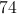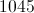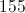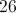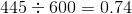ISEE Middle Level Math : How to find percentage

Example Questions

← Previous 1 3 4 5 6 7 8 9 16 17

Example Question #1 : Percentage

A watch originally cost $55.00. If it is on sale for 20% off, what is the sale price of the watch? Possible Answers: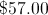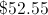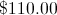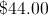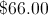Correct answer:Explanation: To solve: First, find what is 20% of 55 by multiplying: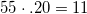Then, subtract 20% (11) from the original price: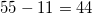The final sale price will be$44.00.

Example Question #2 : Percentage

Which is largest?

a. 30% of 150

b. 20% of 300

c. 40% of 60

d. 10% of 400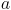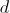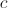Explanation:

To solve:

First find the corresponding percentage of each multiple choice answer,

a)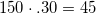b)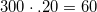c)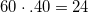d)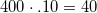Then, compare the amounts and select which amount is the largest:

b) 60

Example Question #3 : Percentage

How do you writeas a percent?

25%

20%

50%

15%

5%

20%

Explanation:is equal to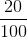, which is 20%.

Example Question #4 : Percentage

Andrew has completed 80% of the race. If the race is 5000 meters, how far along is Andrew?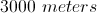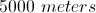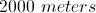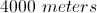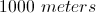Explanation:

Multiply the length of the race by the percentage accomplished by Andrew: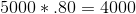Answer: Andrew has completed 4000 meters of the race.

Example Question #5 : Percentage

Which is less?

a. 60% of 10

b. 20% of 40

c. 30% of 20

d. 10% of 50Explanation:

Find the amounts by multiplying each number to the percentage rate: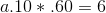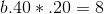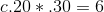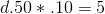Compare the amounts to find the least. The answer is D.

Example Question #6 : Percentage

A round trip flight is on sale for $425.00. If the flight originally costs$500.00, what percentage was it reduced by?Explanation:

Subtract the sale price from the original price: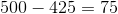Divide that amount by the original price to find the percentage of the sale.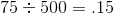Answer: The flight was reduced by 15%.

Example Question #7 : Percentage

Anita is sewing a quilt made of 120 patches. She has sewn together 84 patches already. What percentage of the quilt has Anita sewn?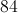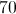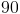Explanation:

Divide 84 by the entire amount of patches 120 to find the percentage completed.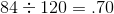The answer is Anita has completed 70% of the quilt.

Example Question #8 : Percentage

Phil filled up 25 balloons for a party. By the end of the night, only 15 balloons were left; the rest had popped. What percentage of balloons popped throughout the party?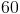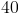Explanation:

Subtract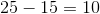to find the amount of balloons that popped.

Then, divide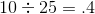The answer: 40% of the balloons popped.

Example Question #9 : Percentage

A pair of sneakers is on sale for 20% off the original price of $120.00. What is the sale price of the sneakers? Possible Answers: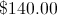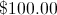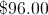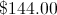Correct answer:Explanation: First, multiply 120 by 0.20.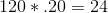Subtract that amount from the original price: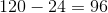Answer: The sneaker's sale price is$96.00

Example Question #10 : Percentage

Carolina needs to complete a 600-word essay for a test she is taking. If she has already written 445 words, what percentage of the essay has she completed? (Round to the nearest percent.)